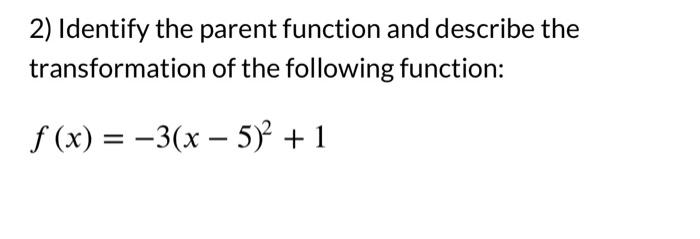Home / Expert Answers / Precalculus / 2-identify-the-parent-function-and-describe-the-transformation-of-the-following-function-f-x-pa692

# (Solved): 2) Identify the parent function and describe the transformation of the following function: \[ f(x)= ...2) Identify the parent function and describe the transformation of the following function: \[ f(x)=-3(x-5)^{2}+1 \]

We have an Answer from Expert